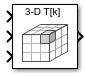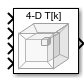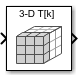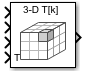Documentation

This is machine translation

Mouseover text to see original. Click the button below to return to the English version of the page.

Direct Lookup Table (n-D)

Index into n-dimensional table to retrieve element, vector, or 2-D matrix

• Library:

HDL Coder / Lookup TablesDescription

The Direct Lookup Table (n-D) block indexes into an n-dimensional table to retrieve an element, vector, or 2-D matrix. The first selection index corresponds to the top (or left) input port. You can choose to provide the table data as an input to the block, or define the table data on the block dialog box. The number of input ports and the size of the output depend on the number of table dimensions and the output slice you select.

If you select a vector from a 2-D table, the output vector can be a column or a row, depending on the model configuration parameter setting Math and Data Types > Use algorithms optimized for row-major array layout. The block inputs are zero-based indices (for more information, see the Inputs select this object from table parameter.

The Direct Lookup Table block supports symbolic dimensions.

Block Inputs and Outputs

The Direct Lookup Table (n-D) block uses inputs as zero-based indices into an n-dimensional table. The number of inputs varies with the shape of the output: an element, vector, or 2-D matrix.

You define a set of output values as the Table data parameter. For the default column-major algorithm behavior, the first input specifies the zero-based index to the table dimension that is one higher than the output dimensionality. The next input specifies the zero-based index to the next table dimension, and so on.

Output ShapeOutput DimensionalityTable Dimension that Maps to the First Input
Element01
Vector12
Matrix23

Suppose that you want to select a vector of values from a 4-D table.The following mapping of block input port to table dimension applies.

This input port...Is the index for this table dimension...
12
23
34

Changes in Block Icon Appearance

Depending on parameters you set, the block icon changes appearance. For table dimensions higher than 4, the icon matches the 4-D version but shows the exact number of dimensions at the top.

When you use the Table data parameter, you see these icons for the default column-major behavior. Some icons are different when you select the configuration parameter Math and Data Types > Use algorithms optimized for row-major array layout.

Object that Inputs Select from the TableNumber of Table Dimensions
1234

ElementVector2-D Matrix

Not applicableWhen you use the table input port, you see these icons.

Object that Inputs Select from the TableNumber of Table Dimensions
1234

ElementVector2-D Matrix

Not applicablePorts

Input

expand all

For the default column-major algorithm, the first input port, specifying the zero-based index to the table dimension that is one higher than the output dimensionality (0, 1, or 2). The next input specifies the zero-based index to the next table dimension, and so on. All index inputs must be real-valued.

Data Types: `single` | `double` | `int8` | `int16` | `int32` | `uint8` | `uint16` | `uint32` | `Boolean` | `fixed point` | `enumerated`

For the default column-major algorithm, the N-th input port, specifying the zero-based index to the table dimension that is N higher than the output dimensionality (0, 1, or 2). The number of inputs varies with the shape of the output. All index inputs must be real-valued.

Data Types: `single` | `double` | `int8` | `int16` | `int32` | `uint8` | `uint16` | `uint32` | `Boolean` | `fixed point` | `enumerated`

Table data, specified as a vector, matrix, or N-D array. The table size must match the dimensions of the Number of dimensions parameter. The block's output data type is the same as the table data type.

Dependencies

To enable this port, select the Make table an input check box.

Data Types: `single` | `double` | `int8` | `int16` | `int32` | `uint8` | `uint16` | `uint32` | `Boolean` | `fixed point` | `enumerated`

Output

expand all

Output slice, provided as a scalar, vector, or 2-D matrix. The size of the block output is determined by the setting of the Inputs select this object from table parameter. The output data type is the same as the table data type.

Data Types: `single` | `double` | `int8` | `int16` | `int32` | `uint8` | `uint16` | `uint32` | `Boolean` | `fixed point` | `enumerated`

Parameters

expand all

Main

Table

Number of dimensions that the Table data parameter must have. This value determines the number of independent variables for the table and the number of inputs to the block.

To specify...Do this...

1, 2, 3, or 4

Select the value from the drop-down list.

A higher number of table dimensions

Enter a positive integer directly in the field.

The maximum number of table dimensions that this block supports is 30.

Programmatic Use

 Block Parameter: `NumberOfTableDimensions` Type: character vector Values:``` '1' | '2' | '3' | '4' | ... |'30'|``` Default: `'2'`

Select this check box to provide table data to the Direct Lookup Table (n-D) block as a block input. When you select this check box, a new input port, `T`, appears. Use this port to input the table data.

Programmatic Use

 Block Parameter: `TableIsInput` Type: character vector Values:``` 'off' | 'on'``` Default: `'off'`

Specify the table of output values. The table size must match the dimensions of the Number of table dimensions parameter.

Tip

During block diagram editing, you can leave the Table data field empty. But for simulation, you must match the number of dimensions in Table data to the Number of table dimensions. For details on how to construct multidimensional MATLAB® arrays, see Multidimensional Arrays (MATLAB).

Click to open the Lookup Table Editor. For more information, see Edit Lookup Tables.

Dependencies

To enable the Table data field, clear the Make table an input check box.

Programmatic Use

 Block Parameter: `Table` Type: character vector Values: `scalar, vector, matrix, or N-D array` Default: `'[4 5 6;16 19 20;10 18 23]'`

Algorithm

Specify whether the output data is a single element, a vector, or a 2-D matrix. The number of input ports for indexing depends on your selection.

SelectionNumber of Input Ports for Indexing
`Element` Number of table dimensions
`Vector` Number of table dimensions -1
`2-D Matrix` Number of table dimensions -2

This numbering matches MATLAB indexing. For example, if you have a 4-D table of data, follow these guidelines.

To access...Specify...As in...
An elementFour indices `array(1,2,3,4)`
A vectorThree indices `array(:,2,3,4)` (default column-major algorithm)
A 2-D matrixTwo indices `array(:,:,3,4)` (default column-major algorithm)

Tips

When the Math and Data Types > Use algorithms optimized for row-major array layout configuration parameter is set, the Direct Lookup Table block behavior changes from column-major to row-major. For this block, the column-major and row-major algorithms may differ semantically in output calculations, resulting in different numerical values. This capability requires a Simulink® Coder™ or Embedded Coder® license. For example, assume that Inputs select this object from table parameter is set to `Vector`. The elements of the selected vector are contiguous in the table storage memory. This table shows the column-major and row-major algorithm depending on the table dimension:

Table DimensionColumn-Major AlgorithmRow-Major Algorithm
2-D tableColumn vector is selectedRow vector is selected
3-D and higher tableOutput vector is selected from the first dimension of the tableOutput vector is selected from the last dimension of the table

Consider the row-major and column-major direct lookup algorithms with vector output from a 3-D table. The last dimension is the third dimension of a 3-D table. Due to semantic changes, column-major and row-major direct lookup may output different vector size and numerical values.

This figure shows a Direct Lookup Table (n-D) block configured with a 3-D table and a vector output. When the model that contains this block is configured for column-major layout, the block icon shows the column-major algorithm.To have the same block use the row-major algorithm, change the Use algorithms optimized for row-major array layout configuration parameter of the model and recompile. The block icon changes to reflect the change to the algorithm optimized for row-major layout.For more information on row-major support, see Row-Major Array Layout: Simplify integration with external C/C++ code for Lookup Table and other blocks (Simulink Coder).

Programmatic Use

 Block Parameter: `InputsSelectThisObjectFromTable` Type: character vector Values: `'Element' | 'Vector' | '2-D Matrix'` Default: `'Element'`

Specify whether to show a warning or error when an index is out of range with respect to the table dimension. Options include:

• `None` — Produce no response.

• `Warning` — Display a warning and continue the simulation.

• `Error` — Terminate the simulation and display an error.

When you select `None` or `Warning`, the block clamps out-of-range indices to fit table dimensions. For example, if the specified index is 5.3 and the maximum index for that table dimension is 4, the block clamps the index to 4.

Programmatic Use

 Block Parameter: `DiagnosticForOutOfRangeInput` Type: character vector Values: `'None' | 'Warning' | 'Error'` Default: `'Warning'`

Specify the sample time as a value other than -1. For more information, see Specify Sample Time.

Dependencies

This parameter is not visible unless it is explicitly set to a value other than `-1`. To learn more, see Blocks for Which Sample Time Is Not Recommended.

Programmatic Use

 Block Parameter: `SampleTime` Type: character vector Values: scalar Default: `'-1'`

Note

The parameters in the Table Attributes pane are not available if you select Make table an input. In this case, the block inherits all table attributes from the input port with the label `T`.

Specify the minimum value for table data. The default value is `[]` (unspecified).

Programmatic Use

 Block Parameter: `TableMin` Type: character vector Values: scalar Default: `'[]'`

Specify the maximum value for table data. The default value is `[]` (unspecified).

Programmatic Use

 Block Parameter: `TableMax` Type: character vector Values: scalar Default: `'[]'`

Specify the table data type. You can set it to:

• A rule that inherits a data type, for example, ```Inherit: Inherit from 'Table data'```

• The name of a built-in data type, for example, `single`

• The name of a data type class, for example, an enumerated data type class

• An expression that evaluates to a data type, for example, `fixdt(1,16,0)`

Click the buttonto display the Data Type Assistant, which helps you set the data type attributes. For more information, see Specify Data Types Using Data Type Assistant.

Programmatic Use

 Block Parameter: `TableDataTypeStr` Type: character vector Values: ```'Inherit: Inherit from 'Table data'' | 'double' | 'single' | 'int8' | 'uint8' | 'int16' | 'uint16' | 'int32' | 'uint32' | 'boolean' | 'fixdt(1,16)' | 'fixdt(1,16,0)' | 'fixdt(1,16,2^0,0)'|'Enum: '|''``` Default: `'Inherit: Inherit from 'Table data''`

Select this parameter to prevent the fixed-point tools from overriding the data types you specify on this block. For more information, see Lock the Output Data Type Setting (Fixed-Point Designer).

Programmatic Use

 Block Parameter: `LockScale` Type: character vector Values: `'off' | 'on'` Default: `'off'`

Block Characteristics

 Data Types `Boolean` | `double` | `enumerated` | `fixed point[a]` | `integer` | `single` Direct Feedthrough `yes` Multidimensional Signals `yes` Variable-Size Signals `no` Zero-Crossing Detection `no` [a] This block supports fixed-point data types for 'Table' data only.